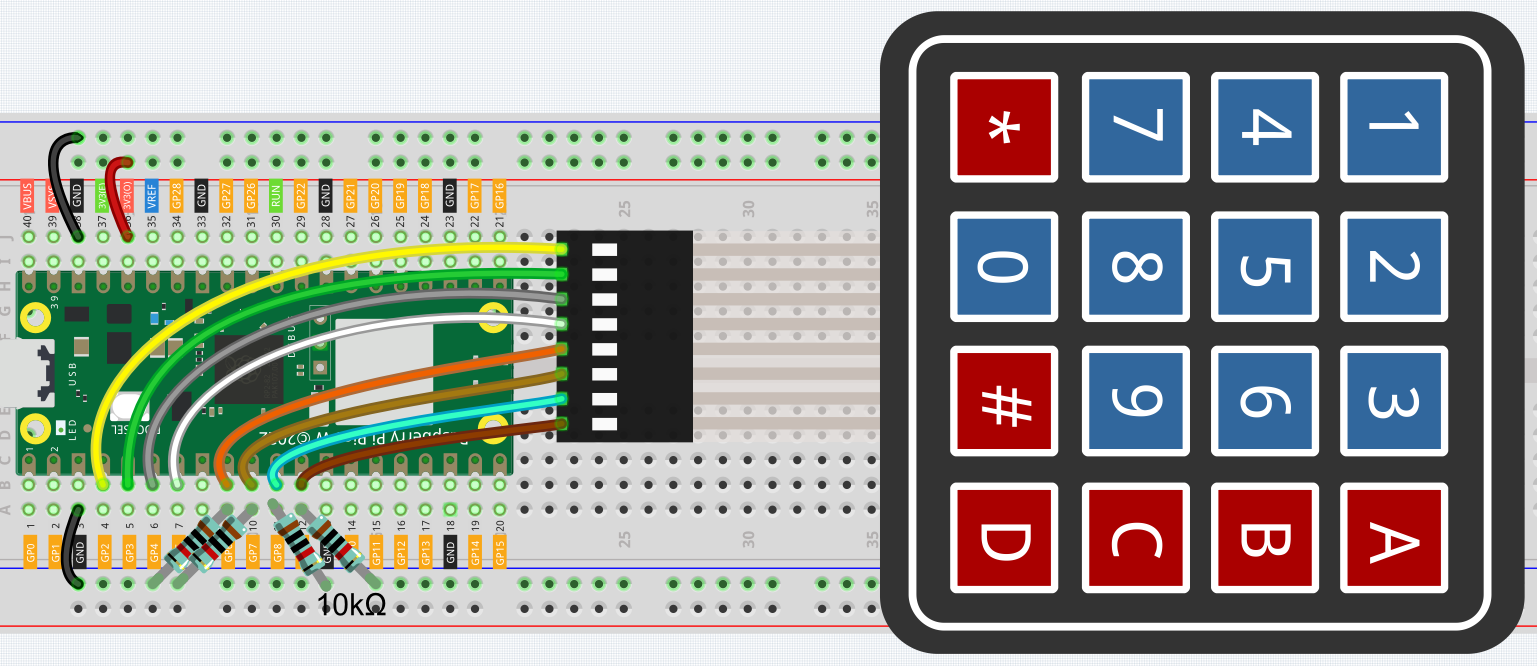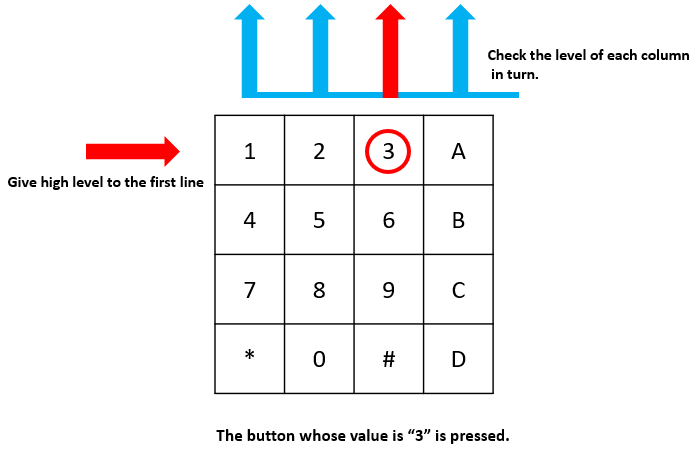The 4x4 keyboard, also known as the matrix keyboard, is a matrix of 16 keys excluded in a single panel.

The keypad can be found on devices that mainly require digital input, such as calculators, TV remote controls, push-button phones, vending machines, ATMs, combination locks, and digital door locks.

In this project, we will learn how to determine which key is pressed and get the related key value.

Required Components

In this project, we need the following components.

Name

ITEMS IN THIS KIT

Kepler Kit

450+

Kepler Kit

SN

COMPONENT

QUANTITY

1

Raspberry Pi Pico W

1

2

Micro USB Cable

1

3

1

4

Jumper Wires

Several

5

Resistor

4(10KΩ)

6

1

Schematic4 pull-down resistors are connected to each of the columns of the matrix keyboard, so that G6 ~ G9 get a stable low level when the keys are not pressed.

The rows of the keyboard (G2 ~ G5) are programmed to go high; if one of G6 ~ G9 is read high, then we know which key is pressed.

For example, if G6 is read high, then numeric key 1 is pressed; this is because the control pins of numeric key 1 are G2 and G6, when numeric key 1 is pressed, G2 and G6 will be connected together and G6 is also high.

WiringTo make the wiring easier, in the above diagram, the column row of the matrix keyboard and the 10K resistors are inserted into the holes where G6 ~ G9 are located at the same time.

Code

Note

• Open the `4.2_4x4_keypad.py` file under the path of `kepler-kit-main/micropython` or copy this code into Thonny, then click “Run Current Script” or simply press F5 to run it.

• Don’t forget to click on the “MicroPython (Raspberry Pi Pico)” interpreter in the bottom right corner.

• For detailed tutorials, please refer to Open and Run Code Directly.

```import machine
import time

characters = [["1","2","3","A"],["4","5","6","B"],["7","8","9","C"],["*","0","#","D"]]

pin = [2,3,4,5]
row = []
for i in range(4):
row.append(None)
row[i] = machine.Pin(pin[i], machine.Pin.OUT)

pin = [6,7,8,9]
col = []
for i in range(4):
col.append(None)
col[i] = machine.Pin(pin[i], machine.Pin.IN)

key = []
for i in range(4):
row[i].high()
for j in range(4):
if(col[j].value() == 1):
key.append(characters[i][j])
row[i].low()
if key == [] :
return None
else:
return key

last_key = None
while True:
if current_key == last_key:
continue
last_key = current_key
if current_key != None:
print(current_key)
time.sleep(0.1)
```

After the program runs, the Shell will print out the keys you pressed on the Keypad.

How it works

```import machine
import time

characters = [["1","2","3","A"],["4","5","6","B"],["7","8","9","C"],["*","0","#","D"]]

pin = [2,3,4,5]
row = []
for i in range(4):
row.append(None)
row[i] = machine.Pin(pin[i], machine.Pin.OUT)

pin = [6,7,8,9]
col = []
for i in range(4):
col.append(None)
col[i] = machine.Pin(pin[i], machine.Pin.IN)
```

Declare each key of the matrix keyboard to the array `characters[]` and define the pins on each row and column.

```last_key = None
while True:
if current_key == last_key:
continue
last_key = current_key
if current_key != None:
print(current_key)
time.sleep(0.1)
```

This is the part of the main function that reads and prints the button value.

The function `readKey()` will read the state of every button.

The statement `if current_key != None` and `if current_key == last_key` is used to judge whether a key is pressed and the state of the pressed button. (If you press '3' when you press '1', the judgement is tenable.)

Prints the value of the currently pressed key when the condition is tenable.

The statement `last_key = current_key` assigns the state of each judgment to an array `last_key` to facilitate the next round of conditional judgment.

```def readKey():
key = []
for i in range(4):
row[i].high()
for j in range(4):
if(col[j].value() == 1):
key.append(characters[i][j])
row[i].low()
if key == [] :
return None
else:
return key
```

This function assigns a high level to each row in turn, and when the button is pressed, the column in which the key is located gets a high level. After the two-layer loop is judged, the value of the button whose state is 1 is stored in the array `key` .

If you press the key '3':`row` is written in high level, and `col` gets high level.

`col`, `col`, `col` get low level.

There are four states:0, 0, 1, 0; and we write '3' into `pressed_keys`.

When `row` , `row` , `row` are written into high level, `col` ~ `col` get low level.

The loop stopped, there returns key = '3'.

If you press the buttons '1' and '3', there will return key = ['1','3'].